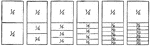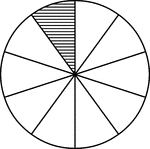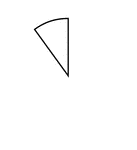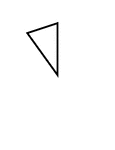### Fraction Comparisons

Rectangles comparing fraction values to one half: 1/4, 1/6, 1/8, 1/10, and 1/12.### Numerical fraction 1/10

Numerical fraction 1/10### Fraction Pie Divided into Tenths

A circle divided into tenths with one tenth shaded.### One Tenhs of a Fraction Pie

One tenth of a circle.### Fractions of 10-sided Polygon

1/10 of a 10 sided polygon